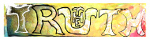发现 ms@ms.lt +370 607 27 665 My work is in the Public Domain for all to share freely. Software Submitted for the workshop Logic and Physics at the 6th World Congress on Universal Logic, June 21-26, Vichy, France. Relating Metaphysics, Logic, Math and Physics with a Taxonomy of Duality We distinguish various notions of duality and explore their significance for understanding and relating metaphysics, logic, math and physics. Metaphysically, we document cognitive frameworks which our minds use to consider fundamental issues from a variety of perspectives. In contemplating existence, we need two perspectives: one to question, whether or not a chair exists (opposites coexist, as in free will), and another to answer definitively (it is how it is, as with fate). Participation evokes three perspectives: taking a stand, following through, and reflecting. Knowedge depends on four perspectives: whether, what, how and why. We show how the logical square makes use of seven perspectives - four corners and three sides - in order to perfectly balance two opposing points of view, without prejudice, such as thinking in terms of "that which is" and "that which is not", but more generally, to balance our unconscious knowledge and our conscious ignorance, as stereotypically championed by the right and left hemispheres of our brains, or by female and male gender roles. We thus argue that the purpose of logic is to allow us to think formally, uninfluenced by content, and this requires the duality which we observe in De Morgan's laws, by which the negation of a disjunction is the conjunction of the negations. This same duality appears in set theory, as linked by Stone's representation theorem. In this way, we can imagine set theory as central to mathematics, and then explore how various branches of mathematics deviate from this duality. For example, topology is dictated by open sets being generated by arbitrary unions of open sets, but only finite intersections. We then investigate mathematics by categorizing more than 60 dualities listed in Wikipedia, the nLab wiki, and Math Overflow. Qualitatively, we distinguish six fundamental kinds of duality, which we may term: Conjugation, Halving of space, Bottom-up vs. Top-down, Functionals, Adjunction, Reversing arrows. These six fundamental kinds are organized by an overall Anti-duality which balances internal structure (as permuted by symmetric groups) and external relationships (as manipulated by matrix multiplication). Within this system there are also many Translations which connect one mathematical framework with another, as does Galois theory. The six dualities provide a mathematical map of important theorems and concepts. Conjugation. The two square roots of -1 are completely indistinguishable, a fact which is lost upon naming them +i and -i. This internal duality is important for the complexes, quaternions and octonions. Halving a space, as with root systems. Rotation looks different from the opposite side. Reflection takes us back and forth across a space. Reversing the order of algebraic operations is such a reflection. Bottom-up and Top-down, as with plane duality, projective geometry, homology and Riemann-Roch. The binomial theorem for (x+y)^n holds when we swap x = "what is" and y = "what is not" in a subset. These internal dualities are mirrored by the following external dualities. Reversing arrows. Category theory exults in limits and colimits, etc. as do partially ordered sets. Functionals. An object (like a vector space) can be dual to the maps from it to a trivial space (like a field). This is Grothendieck's relative point of view. Adjunction. Expanding structure by 1->AB is mirrored by collapsing structure XY->1. We sketch out a map of how these dualities relate various branches of mathematics. We then consider what this might suggest for physics. Electricity and magnetism can be considered as conjugates. We remark on S-duality, T-duality, mirror symmetry, and Sir Michael Atiyah's lecture "Duality in Mathematics and Physics". Naujausi pakeitimai Introduction E9F5FC Understandable FFFFFF Questions FFFFC0 Notes EEEEEE 维基百科 影片 书 读物
 Puslapis paskutinį kartą pakeistas 2017 lapkričio 01 d., 09:43